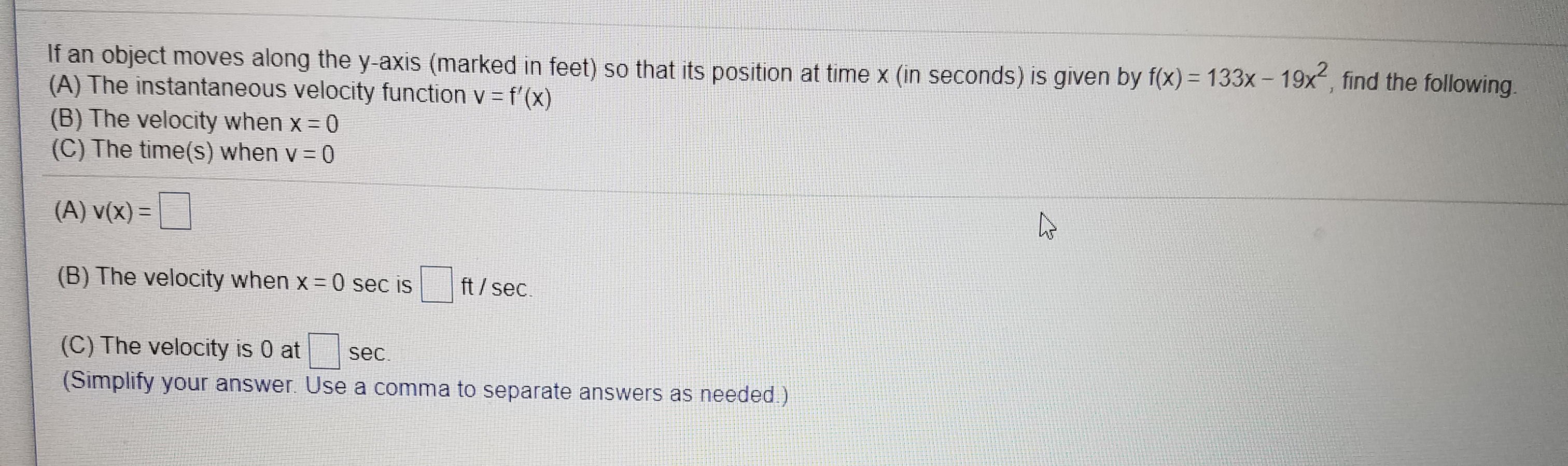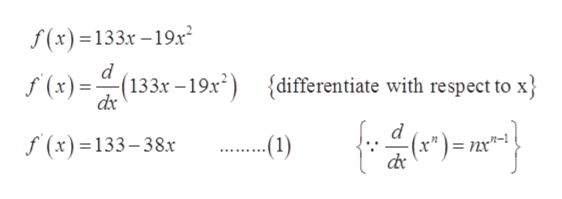If an object moves along the y-axis (marked in feet) so that its position at time x (in seconds) is given by f(x) 133x- 19x-, find the following.(A) The instantaneous velocity function v f' (x)(B) The velocity when x 0(C) The time(s) when v 0(A) v(x)(B) The velocity when x 0 sec isft/sec(C) The velocity is 0 atsec(Simplify your answer. Use a comma to separate answers as needed)

Question

See attachedhelp_outlineImage TranscriptioncloseIf an object moves along the y-axis (marked in feet) so that its position at time x (in seconds) is given by f(x) 133x- 19x-, find the following. (A) The instantaneous velocity function v f' (x) (B) The velocity when x 0 (C) The time(s) when v 0 (A) v(x) (B) The velocity when x 0 sec is ft/sec (C) The velocity is 0 at sec (Simplify your answer. Use a comma to separate answers as needed) fullscreen
Step 1

To determine the following part, mentioned below.

(A). The instantaneous velocity function v=f(x).

(B). The velocity when x=0.

(C). The time(s) when v=0.

Step 2

Given information:

Step 3

Part (A): The instantaneous velocity fun...help_outlineImage Transcriptionclosef(x)=133x -19.x d f (x)(133x-19x") {differentiate with respect to x dx d f (x)=133-38.x -(1) fullscreen

Want to see the full answer?

See Solution

Want to see this answer and more?

Our solutions are written by experts, many with advanced degrees, and available 24/7

See Solution
Tagged in

Derivative